# ICSE Selina Solution for Class 9 Chemistry Chapter 2

## ICSE Selina Solution for Class 9 Chemistry Chapter 2 PDF – Free PDF Download

ICSE Selina Solution for Class 9 Chemistry Chapter 2 Chemical Changes and Reaction is presented here for the benefit of the students. Selina Solution for class 9 Chemistry is prepared by a subject expert after comprehensive research and understanding of the concepts involved in chemical changes and reactions.

ICSE Selina solutions are one of the best study materials that most expert teachers recommend to students studying ICSE Syllabus. Studying it thoroughly will aid you in an understanding the concepts and will build a problem-solving capacity in you.

This ICSE Selina Concise Solution for class 9 chapter 2 supports you in understanding types of chemical changes such as direct combination, decomposition, displacement and double replacement. After studying this study resource, you will also be able to attain knowledge on energy changes in a chemical reaction, meaning and examples of exothermic and endothermic reactions.

• Chemical change
• Characteristics of chemical change
• Types of chemical reactions
• Energy change in chemical reactions
• Photochemical reaction
• Electrochemical reaction

### Download PDF of  ICSE Selina Concise Solution for Class 9 Chemistry Chapter 2 Chemical Changes and Reaction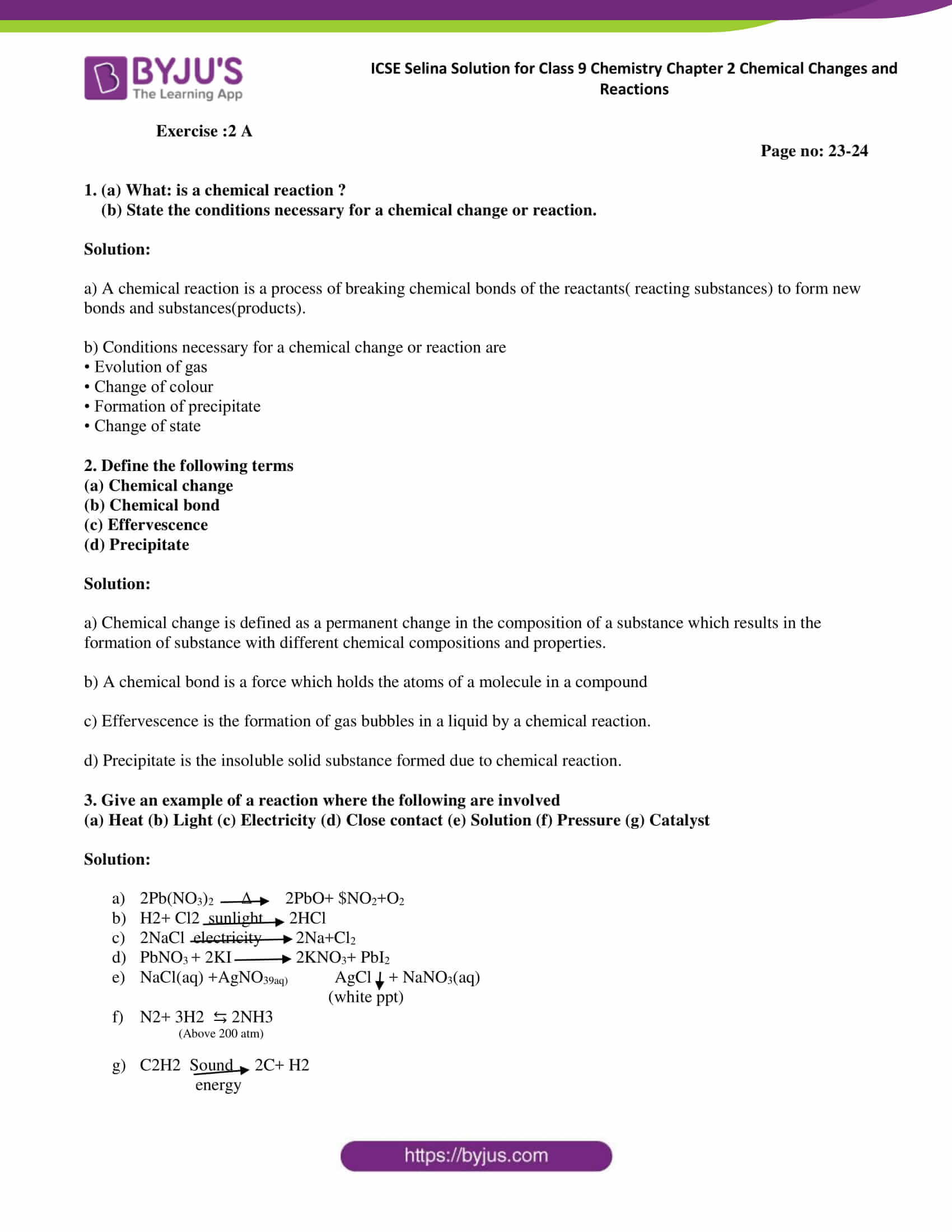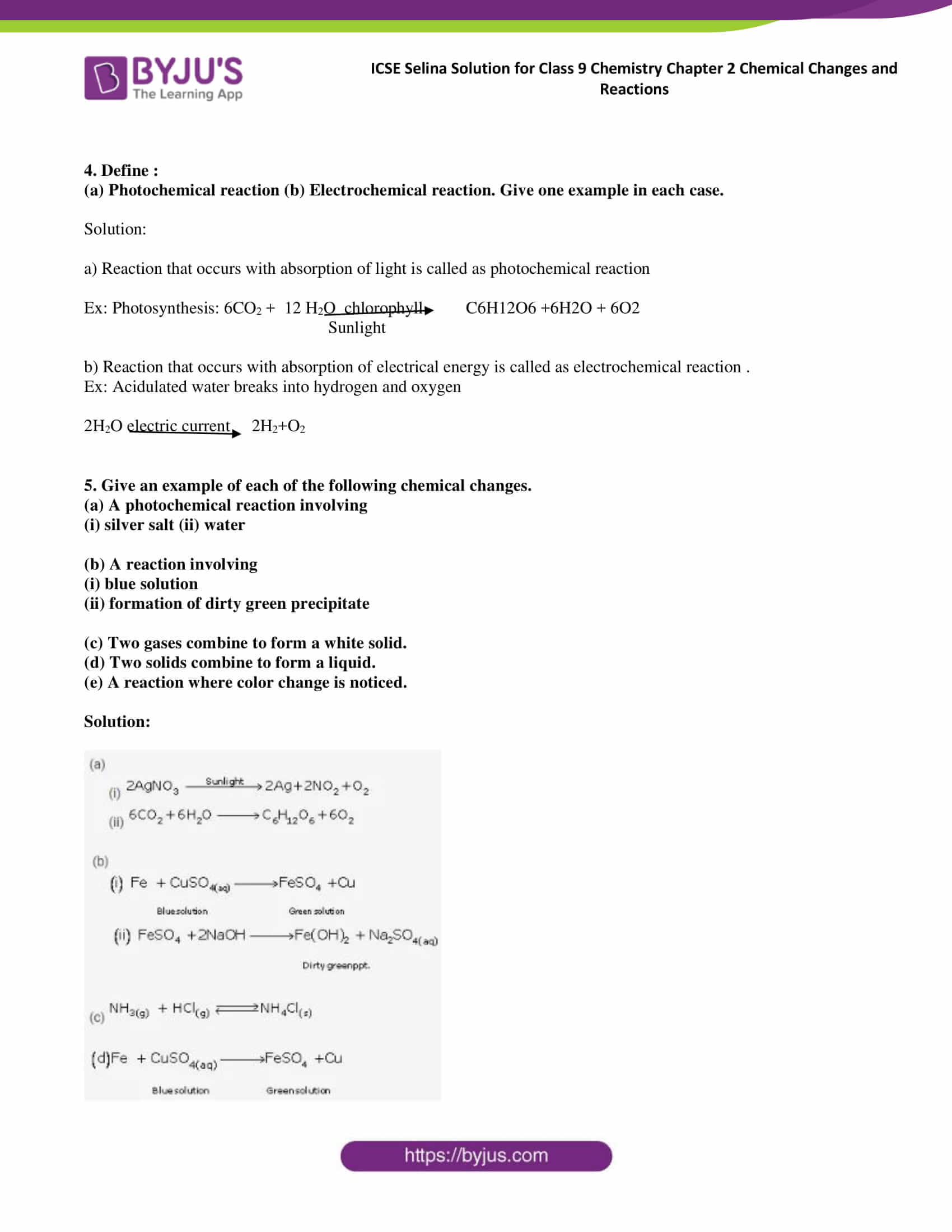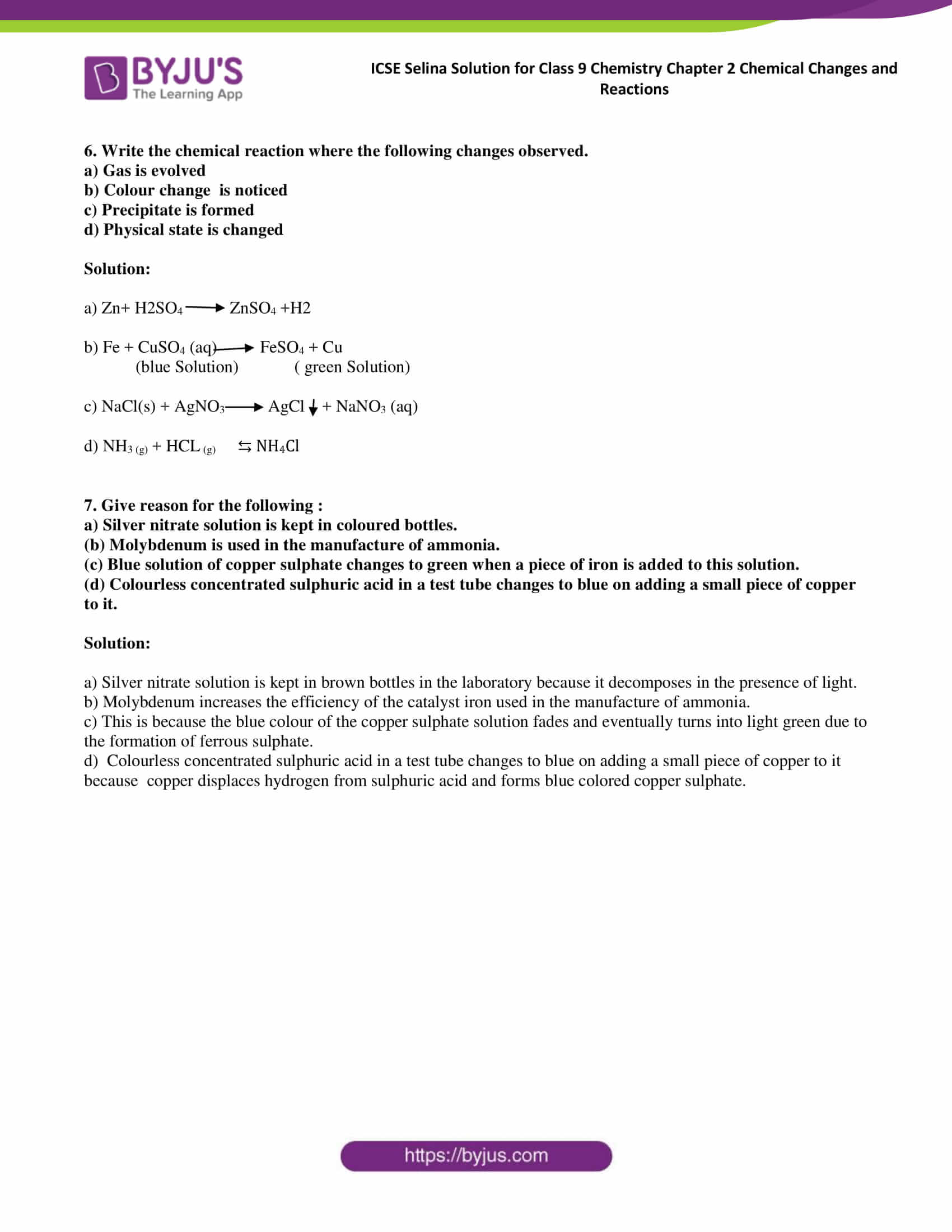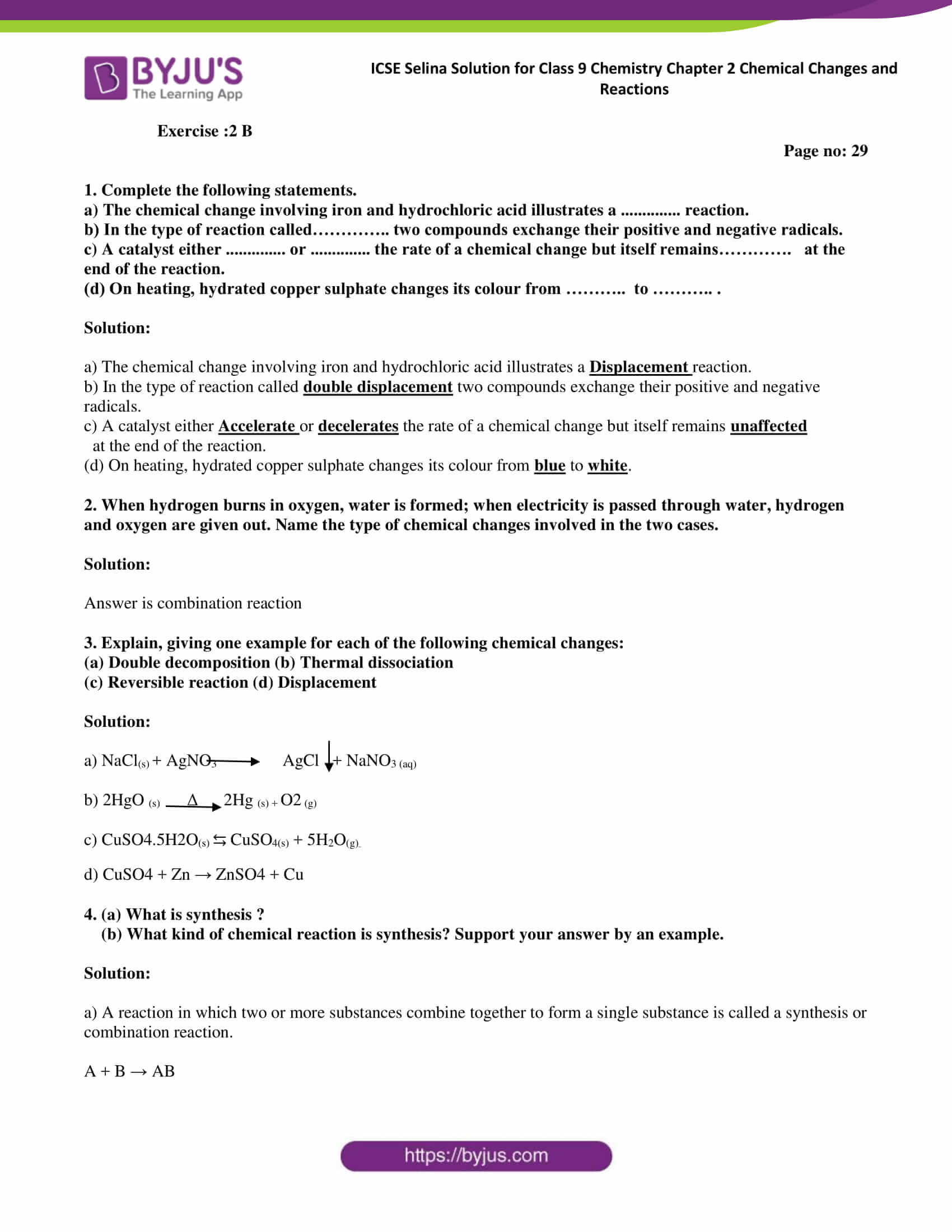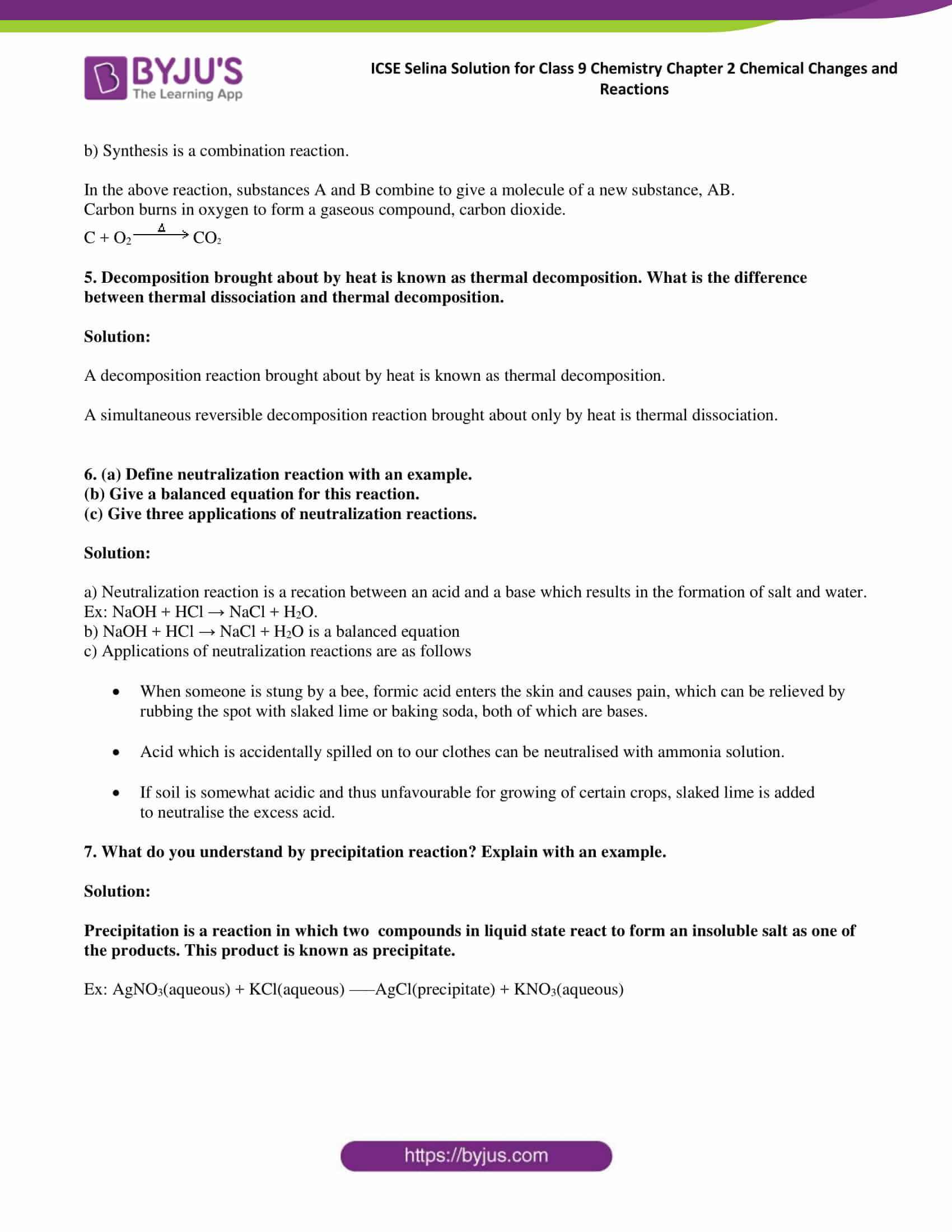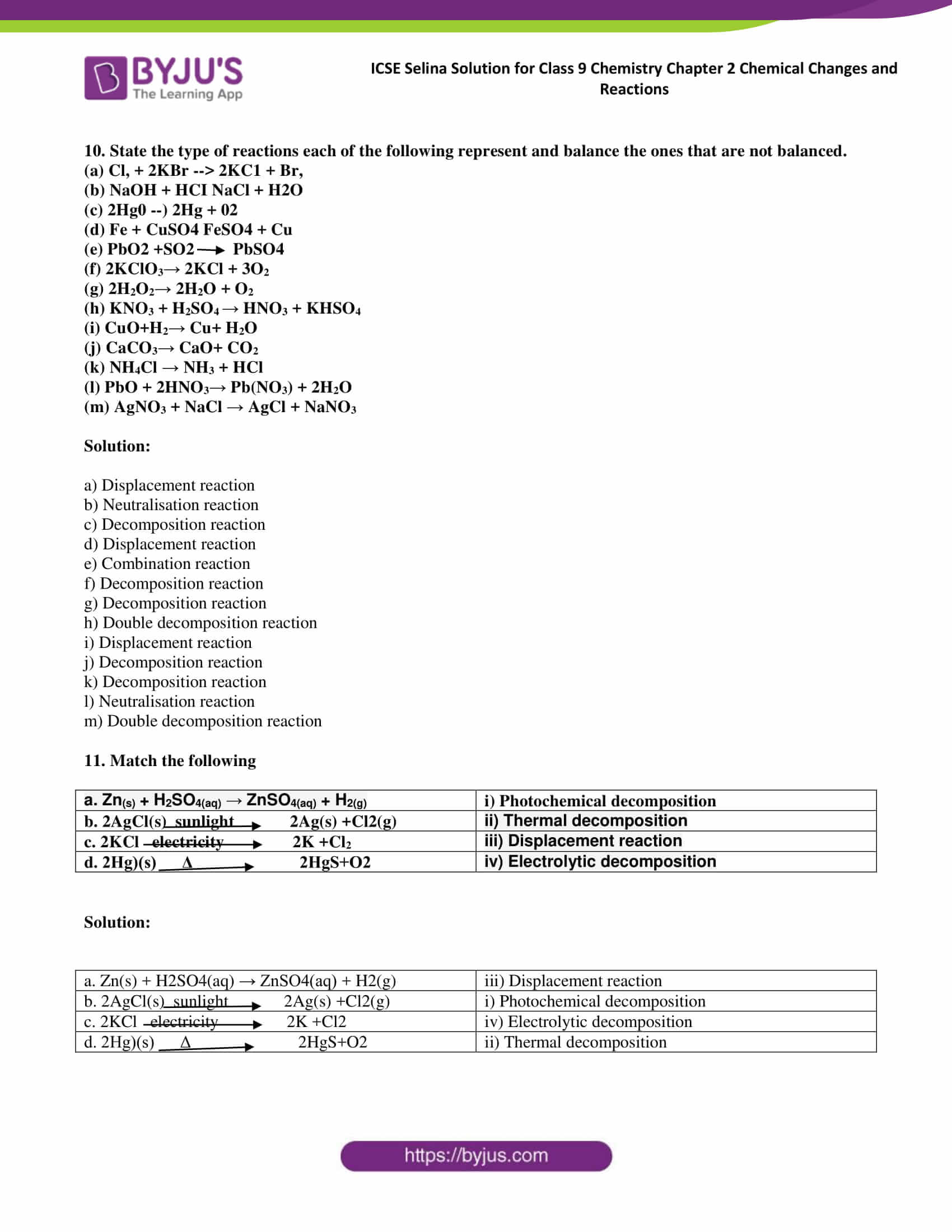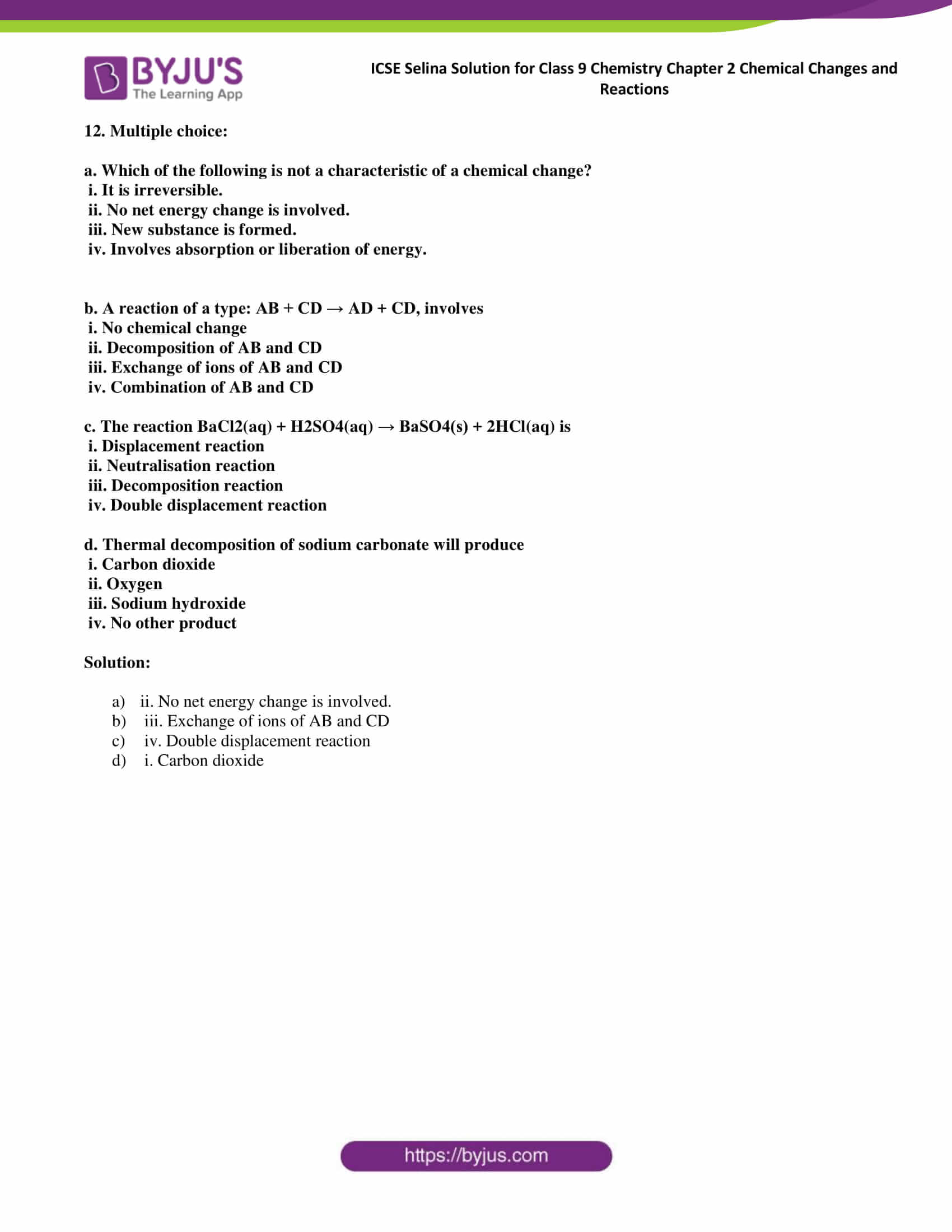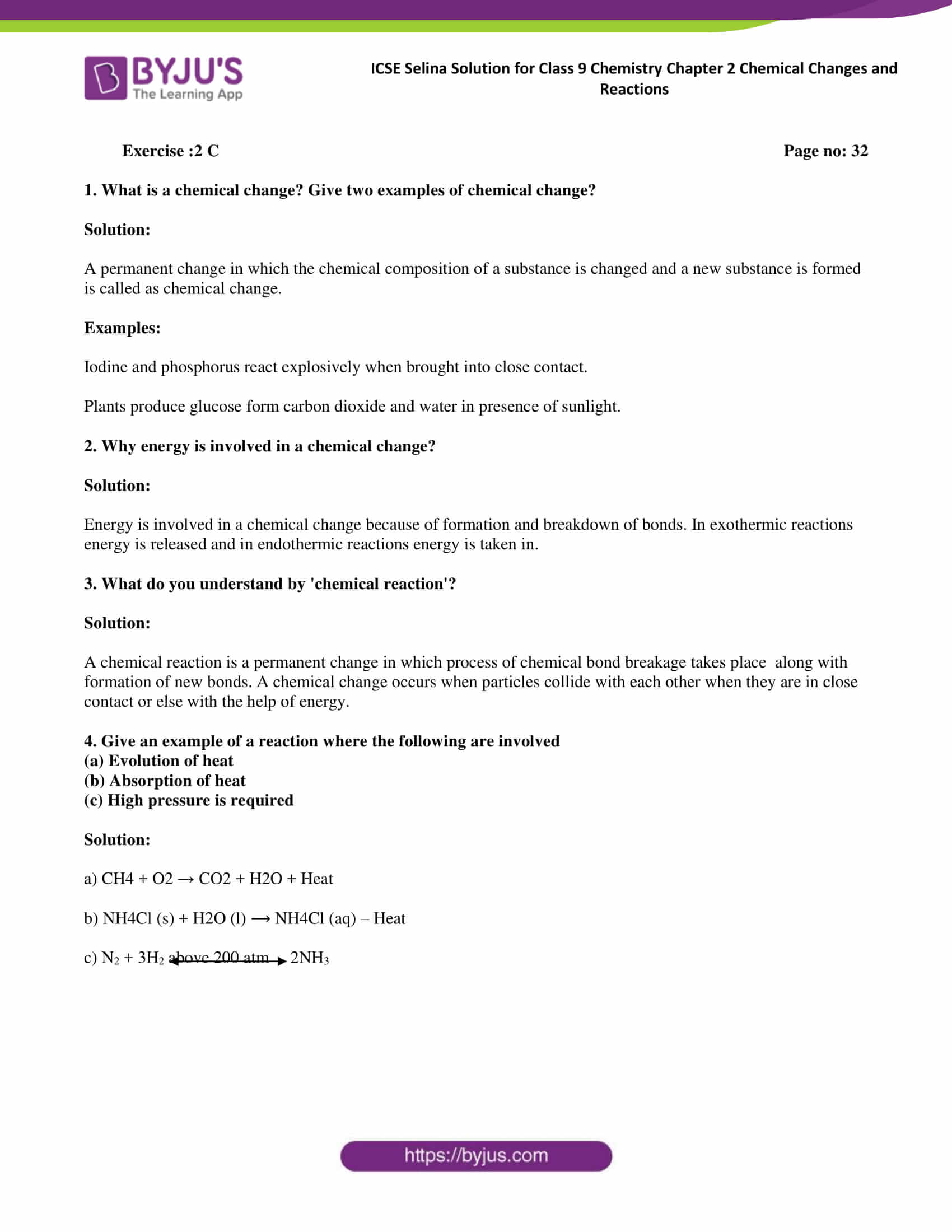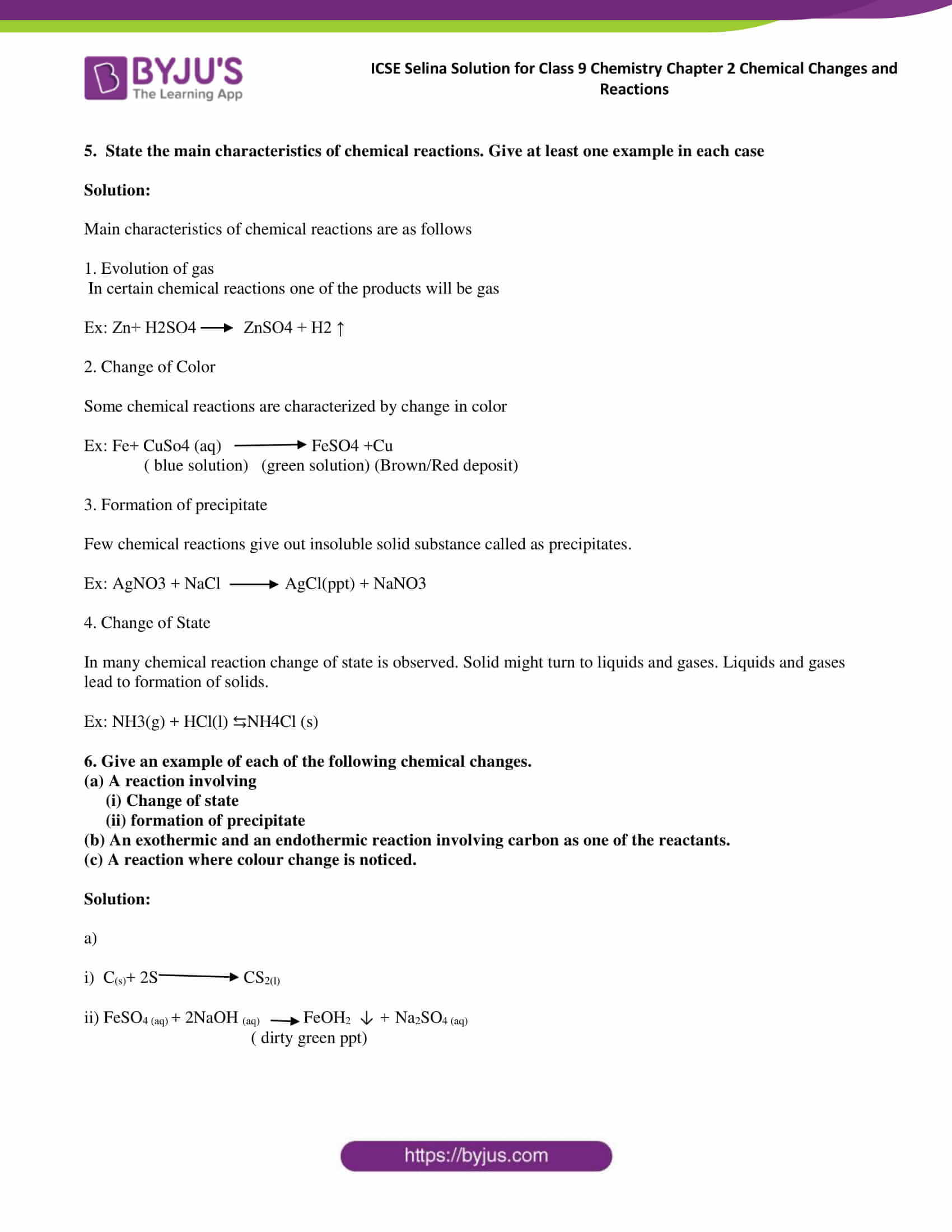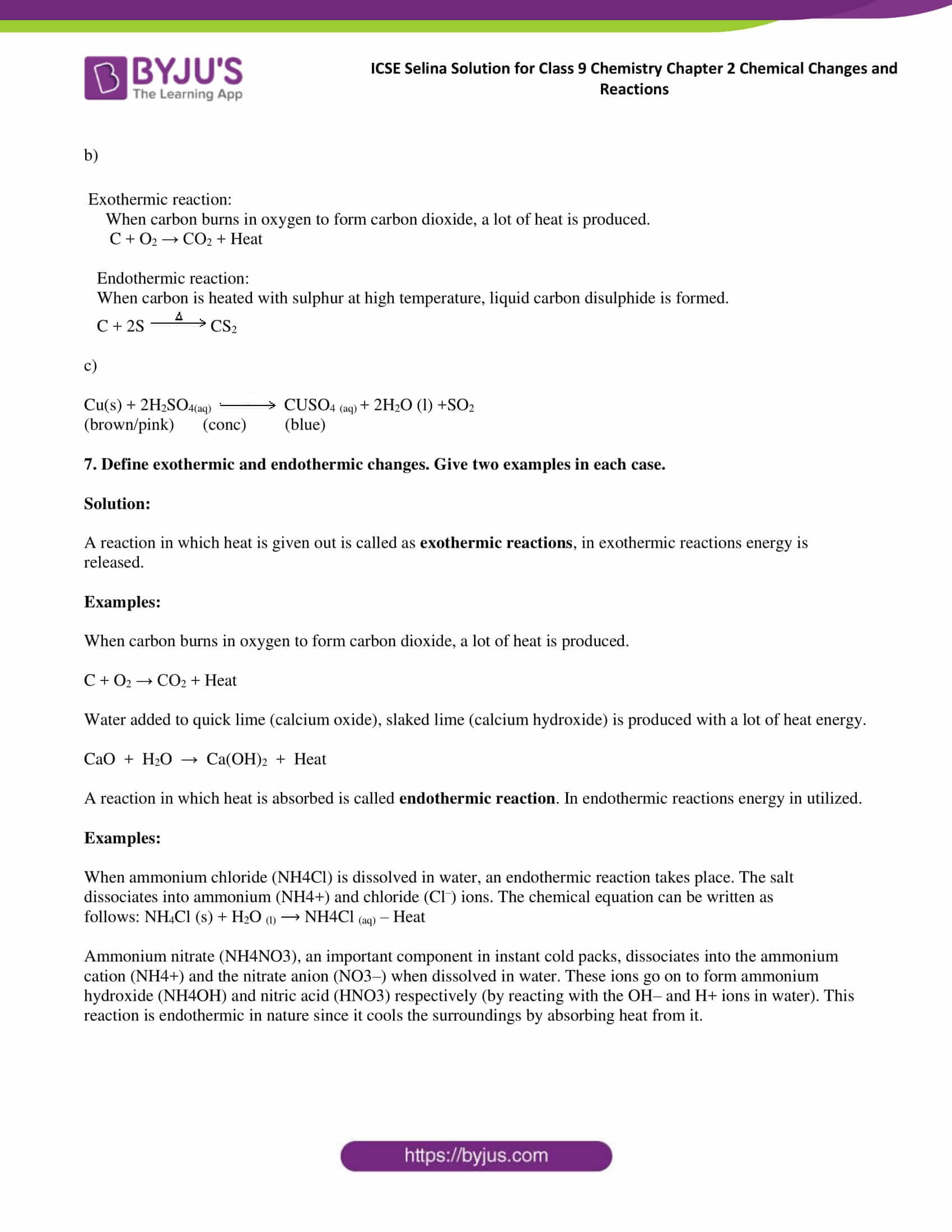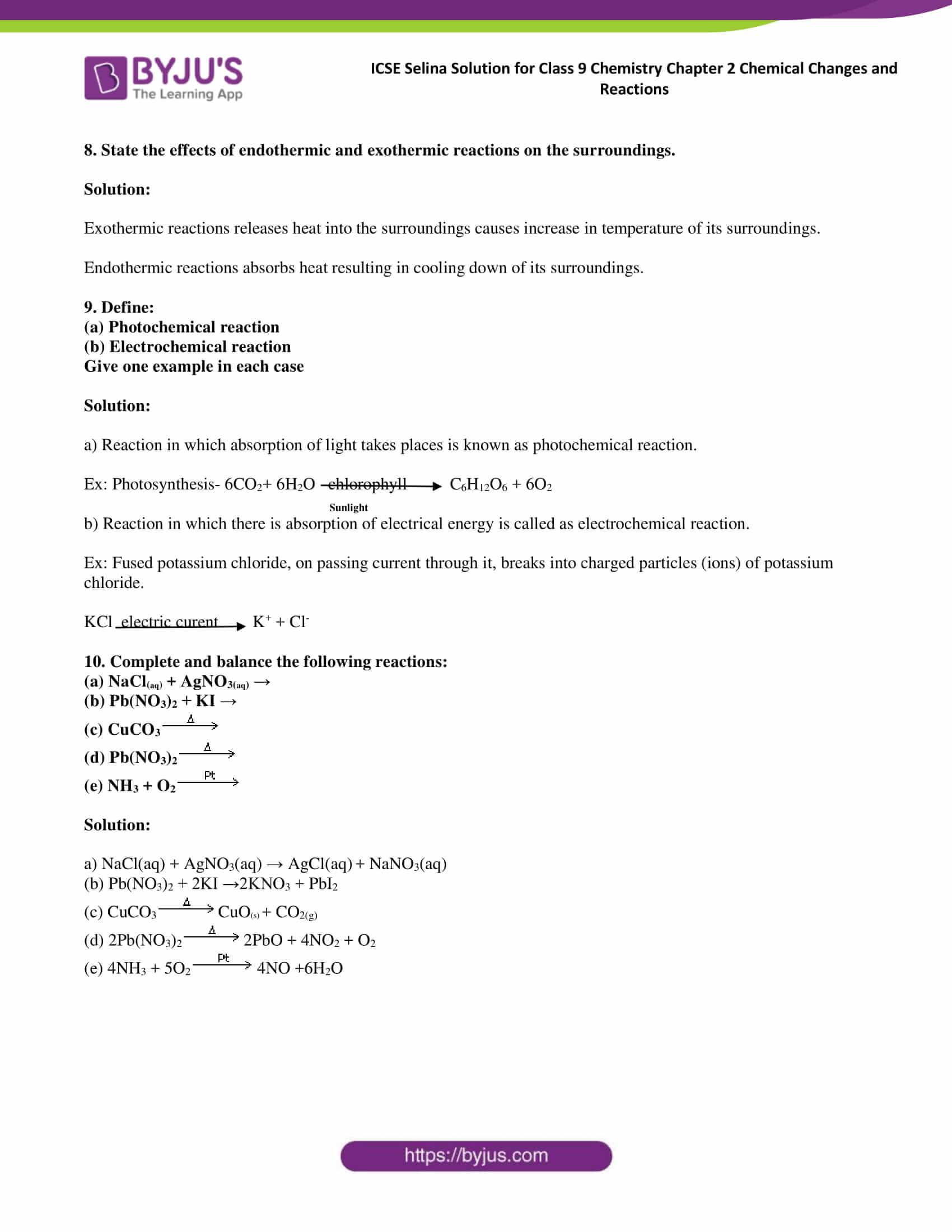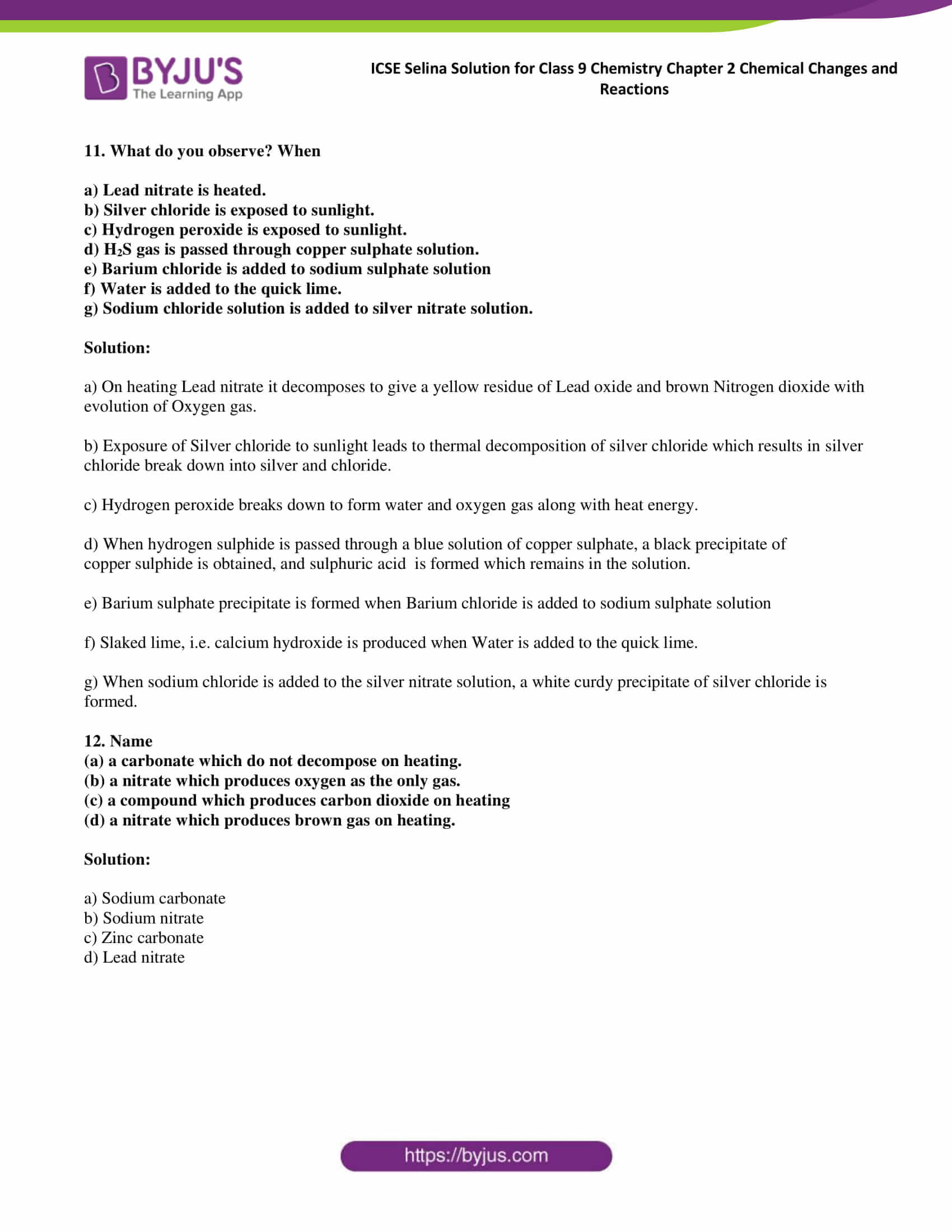### Access answers of Selina publication ICSE Class 9 Chemistry Chapter 2 Chemical Changes and Reaction

Exercise:2

1. (a) What: is a chemical reaction?

(b) State the conditions necessary for a chemical change or reaction.

Solution:

a) A chemical reaction is a process of breaking chemical bonds of the reactants( reacting substances) to form new bonds and substances(products).

b) Conditions necessary for a chemical change or reaction are

• Evolution of gas

• Change of colour

• Formation of a precipitate

• Change of state

2. Define the following terms

(a) Chemical change

(b) Chemical bond

(c) Effervescence

(d) Precipitate

Solution:

a) A chemical change is defined as a permanent change in the composition of a substance which results in the formation of substance with different chemical compositions and properties.

b) A chemical bond is a force which holds the atoms of a molecule in a compound

c) Effervescence is the formation of gas bubbles in a liquid by a chemical reaction.

d) The precipitate is the insoluble solid substance formed due to chemical reaction.

3. Give an example of a reaction where the following are involved

(a) Heat (b) Light (c) Electricity (d) Close contact (e) Solution (f) Pressure (g) Catalyst

Solution: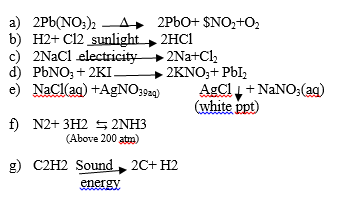4. Define :

(a) Photochemical reaction (b) Electrochemical reaction. Give one example in each case.

Solution:

a) A reaction that occurs with the absorption of light is called a photochemical reaction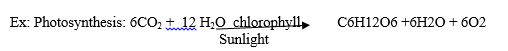b) A reaction that occurs with the absorption of electrical energy is called an electrochemical reaction.

Ex: Acidulated water breaks into hydrogen and oxygen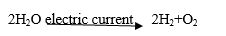5. Give an example of each of the following chemical changes.

(a) A photochemical reaction involving

(i) silver salt (ii) water

(b) A reaction involving

(i) blue solution

(ii) formation of a dirty green precipitate

(c) Two gases combine to form a white solid.

(d) Two solids combine to form a liquid.

(e) A reaction where color change is noticed.

Solution: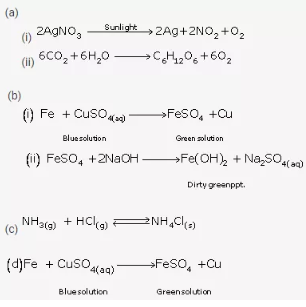6. Write the chemical reaction where the following changes observed.

a) Gas is evolved

b) Colour change is noticed

c) Precipitate is formed

d) Physical state is changed

Solution: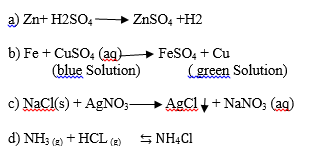7. Give a reason for the following :

a) Silver nitrate solution is kept in coloured bottles.

(b) Molybdenum is used in the manufacture of ammonia.

(c) A blue solution of copper sulphate changes to green when a piece of iron is added to this solution.

(d) Colourless concentrated sulphuric acid in a test tube changes to blue on adding a small piece of copper to it.

Solution:

a) Silver nitrate solution is kept in brown bottles in the laboratory because it decomposes in the presence of light.

b) Molybdenum increases the efficiency of the catalyst iron used in the manufacture of ammonia.

c) This is because of the blue colour of the copper sulphate solution fades and eventually turns into light green due to the formation of ferrous sulphate.

d) Colourless concentrated sulphuric acid in a test tube changes to blue on adding a small piece of copper to it because copper displaces hydrogen from sulphuric acid and forms blue coloured copper sulphate.

Exercise:2 B

1. Complete the following statements.

a) The chemical change involving iron and hydrochloric acid illustrates a ____________ reaction.

b) In the type of reaction called__________two compounds exchange their positive and negative radicals.

c) A catalyst either ______ or _________ the rate of a chemical change but itself remains__________at the end of the reaction.

(d) On heating, hydrated copper sulphate changes its colour from______ to___________

Solution:

a) The chemical change involving iron and hydrochloric acid illustrates a Displacement reaction.

b) In the type of reaction called double displacement, two compounds exchange their positive and negative radicals.

c) A catalyst either Accelerate or decelerates the rate of a chemical change but itself remains unaffected

at the end of the reaction.

(d) On heating, hydrated copper sulphate changes its colour from blue to white.

2. When hydrogen burns in oxygen, water is formed; when electricity is passed through water, hydrogen and oxygen are given out. Name the type of chemical changes involved in the two cases.

Solution:

The answer is a combination reaction

3. Explain, giving one example for each of the following chemical changes:

(a) Double decomposition (b) Thermal dissociation

(c) Reversible reaction (d) Displacement

Solution: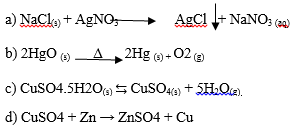4. (a) What is synthesis?

(b) What kind of chemical reaction is synthesis? Support your answer by an example.

Solution:

a) A reaction in which two or more substances combine together to form a single substance is called a synthesis or combination reaction.

A + B → AB

b) Synthesis is a combination reaction.

In the above reaction, substances A and B combine to give a molecule of a new substance, AB.

Carbon burns in oxygen to form a gaseous compound, carbon dioxide.

C + O2

$$\begin{array}{l}\overset{\triangle }{\rightarrow}\end{array}$$
CO2

5. Decomposition brought about by heat is known as thermal decomposition. What is the difference between thermal dissociation and thermal decomposition?

Solution:

A decomposition reaction brought about by heat is known as thermal decomposition.

A simultaneous reversible decomposition reaction brought about only by heat is thermal dissociation.

6. (a) Define neutralization reaction with an example.

(b) Give a balanced equation for this reaction.

(c) Give three applications of neutralization reactions.

Solution:

a) A neutralization reaction is a reaction between an acid and a base which results in the formation of salt and water. Ex: NaOH + HCl → NaCl + H2O.

b) NaOH + HCl → NaCl + H2O is a balanced equation

c) Applications of neutralization reactions are as follows

• When someone is stung by a bee, formic acid enters the skin and causes pain, which can be relieved by rubbing the spot with slaked lime or baking soda, both of which are bases.
• The acid which is accidentally spilt on to our clothes can be neutralised with ammonia solution.
• If soil is somewhat acidic and thus unfavourable for the growth of certain crops, slaked lime is added to neutralise the excess acid.

7. What do you understand by a precipitation reaction? Explain with an example.

Solution:

Precipitation is a reaction in which two compounds in liquid state react to form an insoluble salt as one of the products. This product is known as a precipitate.

Ex: AgNO3(aqueous) + KCl(aqueous) —–AgCl(precipitate) + KNO3(aqueous)

8. (a) What are double displacement reactions? (b) Give an example of a double displacement reaction, where gas is evolved.

Solution:

a) Double displacement reactions are those in which two compounds in a solution react to form two new compounds by mutual exchange of radicals. This type of reaction is also known as a double decomposition reaction.

b) Mg(s) + 2 HCl(aq) → MgCl2(aq) + H2(g)

9. (a) What is a decomposition reaction ?

(b) Decomposition reactions can occur by (i) heat (ii) electricity and (iii) sunlight.

Give two balanced reactions for each.

Solution:

a) A reaction in which a compound splits into two or simpler substances is called decomposition reaction.

b)

i) Heat

CaCO3=CaO+CO2.

FeSO4→ Fe2O3+SO2+SO3

ii) Electricity 2H2O → H2+O2

iii) Light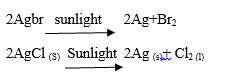10. State the type of reactions each of the following represent and balance the ones that are not balanced.

(a) Cl, + 2KBr → 2KC1 + Br,

(b) NaOH + HCI NaCl + H2O

(c) 2Hg0 –) 2Hg + 02

(d) Fe + CuSO4 FeSO4 + Cu

(e) PbO2 +SO2 PbSO4

(f) 2KClO3→ 2KCl + 3O2

(g) 2H2O2→ 2H2O + O2

(h) KNO3 + H2SO→ HNO3 + KHSO4

(i) CuO+H2→ Cu+ H2

(j) CaCO3→ CaO+ CO

(k) NH4Cl → NH3 + HCl

(l) PbO + 2HNO3→ Pb(NO3) + 2H2

(m) AgNO3 + NaCl → AgCl + NaNO3

Solution:

a) Displacement reaction

b) Neutralisation reaction

c) Decomposition reaction

d) Displacement reaction

e) Combination reaction

f) Decomposition reaction

g) Decomposition reaction

h) Double decomposition reaction

i) Displacement reaction

j) Decomposition reaction

k) Decomposition reaction

l) Neutralisation reaction

m) Double decomposition reaction

11. Match the following

 a. Zn(s) + H2SO4(aq) → ZnSO4(aq) + H2(g) i) Photochemical decomposition b. 2AgCl(s) sunlight → 2Ag(s) +Cl2(g) ii) Thermal decomposition c. 2KCl electricity → 2K +Cl2 iii) Displacement reaction d. 2Hg)(s) Δ → 2HgS+O2 iv) Electrolytic decomposition

Solution:

 a. Zn(s) + H2SO4(aq) → ZnSO4(aq) + H2(g) iii) Displacement reaction b. 2AgCl(s) sunlight 2Ag(s) +Cl2(g) i) Photochemical decomposition c. 2KCl electricity 2K +Cl2 iv) Electrolytic decomposition d. 2Hg)(s) Δ → 2HgS+O2 ii) Thermal decomposition

12. Multiple choice:

a. Which of the following is not a characteristic of a chemical change?

i. It is irreversible.

ii. No net energy change is involved.

iii. New substance is formed.

iv. Involves absorption or liberation of energy.

b. A reaction of a type: AB + CD → AD + CD, involves

i. No chemical change

ii. Decomposition of AB and CD

iii. Exchange of ions of AB and CD

iv. Combination of AB and CD

c. The reaction BaCl2(aq) + H2SO4(aq) → BaSO4(s) + 2HCl(aq) is

i. Displacement reaction

ii. Neutralisation reaction

iii. Decomposition reaction

iv. Double displacement reaction

d. Thermal decomposition of sodium carbonate will produce

i. Carbon dioxide

ii. Oxygen

iii. Sodium hydroxide

iv. No other product

Solution:

1. ii. No net energy change is involved.
2.  iii. Exchange of ions of AB and CD
3.  iv. Double displacement reaction
4.  i. Carbon dioxide

Exercise:2C

1. What is a chemical change? Give two examples of a chemical change?

Solution:

A permanent change in which the chemical composition of a substance is changed and a new substance is formed is called as chemical change.

Examples:

Iodine and phosphorus react explosively when brought into close contact.

Plants produce glucose from carbon dioxide and water in the presence of sunlight.

2. Why energy is involved in a chemical change?

Solution:

Energy is involved in a chemical change because of formation and breakdown of bonds. In exothermic reactions, energy is released and in endothermic reactions, energy is taken in.

3. What do you understand by ‘chemical reaction’?

Solution:

A chemical reaction is a permanent change in which the process of chemical bond breakage takes place along with the formation of new bonds. A chemical change occurs when particles collide with each other when they are in close contact or else with the help of energy.

4. Give an example of a reaction where the following are involved

(a) Evolution of heat

(b) Absorption of heat

(c) High pressure is required

Solution:

a) CH4 + O2 → CO2 + H2O + Heat

b) NH4Cl (s) + H2O (l) ⟶ NH4Cl (aq) – Heat

c) N2 + 3H2 above 200 atm 2NH3

5. State the main characteristics of chemical reactions. Give at least one example in each case

Solution:

Main characteristics of chemical reactions are as follows

1. Evolution of gas

In certain chemical reactions, one of the products will be gas

Ex: Zn+ H2SO4 →ZnSO4 + H2 ↑

2. Change of Color

Some chemical reactions are characterized by a change in colour

Ex: Fe+ CuSo4 (aq) →FeSO4 +Cu

( blue solution) (green solution) (Brown/Red deposit)

3. Formation of precipitate

Few chemical reactions give out an insoluble solid substance called as precipitates.

Ex: AgNO3 + NaCl→ AgCl(ppt) + NaNO3

4. Change of State

In many chemical reaction changes of state is observed. Solid might turn to liquids and gases. Liquids and gases lead to the formation of solids.

Ex: NH3(g) + HCl(l) ⇆NH4Cl (s)

6. Give an example of each of the following chemical changes.

(a) A reaction involving

(i) Change of state

(ii) Formation of a precipitate

(b) An exothermic and an endothermic reaction involving carbon as one of the reactants.

(c) A reaction where the colour change is noticed.

Solution:

a)

i) C(s)+ 2S → CS2(l)

ii) FeSO4 (aq) + 2NaOH (aq)

$$\begin{array}{l}\overrightarrow{dirty \ green \ ppt}\end{array}$$
FeOH2 ↓+Na2SO4 (aq)

b)

Exothermic reaction:

When carbon burns in oxygen to form carbon dioxide, a lot of heat is produced.

C + O2 → CO2 + Heat

Endothermic reaction:

When carbon is heated with sulphur at high temperature, liquid carbon disulphide is formed.

C + 2S

$$\begin{array}{l}\overset{\bigtriangleup }{\rightarrow}\end{array}$$
CS2

c)

Cu(s) + 2H2SO4(aq) →CUSO4 (aq) + 2H2O (l) +SO2

(brown/pink) (conc) (blue)

7. Define exothermic and endothermic changes. Give two examples in each case.

Solution:

A reaction in which heat is given out is called exothermic reactions, in exothermic reactions, energy is released.

Examples:

When carbon burns in oxygen to form carbon dioxide, a lot of heat is produced.

C + O2 → CO2 + Heat

Water added to quick lime (calcium oxide), slaked lime (calcium hydroxide) is produced with a lot of heat energy.

CaO  +  H2O  →  Ca(OH)2  +  Heat

A reaction in which heat is absorbed is called an endothermic reaction. In endothermic reactions, energy is utilized.

Examples:

When ammonium chloride (NH4Cl) is dissolved in water, an endothermic reaction takes place. The salt dissociates into ammonium (NH4+) and chloride (Cl) ions. The chemical equation can be written as

follows: NH4Cl (s) + H2O (l) ⟶ NH4Cl (aq) – Heat

Ammonium nitrate (NH4NO3), an important component in instant cold packs, dissociates into the ammonium cation (NH4+) and the nitrate anion (NO3–) when dissolved in water. These ions go on to form ammonium hydroxide (NH4OH) and nitric acid (HNO3) respectively (by reacting with the OH– and H+ ions in water). This reaction is endothermic in nature since it cools the surroundings by absorbing heat from it.

8. State the effects of endothermic and exothermic reactions on the surroundings.

Solution:

Exothermic reactions release heat into the surroundings causes an increase in the temperature of its surroundings.

Endothermic reactions absorb heat resulting in cooling down of its surroundings.

9. Define:

(a) Photochemical reaction

(b) Electrochemical reaction

Give one example in each case

Solution:

a) A reaction in which absorption of light takes places is known as a photochemical reaction.

Ex: Photosynthesis- 6CO2+ 6H2O chlorophyllsunlight C6H12O6 + 6O2

b) The reaction in which there is the absorption of electrical energy is called an electrochemical reaction.

Ex: Fused potassium chloride, on passing a current through it, breaks into charged particles (ions) of potassium chloride.

KCl

$$\begin{array}{l}\overrightarrow{electric \ current } \end{array}$$
K+ + Cl

10. Complete and balance the following reactions:

(a) NaCl(aq) + AgNO3(aq) →

(b) Pb(NO3)2 + KI →

(c) CuCO3

$$\begin{array}{l}\overset{\bigtriangleup }{\rightarrow}\end{array}$$

(d) Pb(NO3)2

$$\begin{array}{l}\overset{\bigtriangleup }{\rightarrow}\end{array}$$

(e) NH3 + O2

$$\begin{array}{l}\overset{pt }{\rightarrow}\end{array}$$

Solution:

a) NaCl(aq) + AgNO3(aq) → AgCl(aq) + NaNO3(aq)

(b) Pb(NO3)2 + 2KI → 2KNO3 + PbI2

(c) CuCO3

$$\begin{array}{l}\overset{\bigtriangleup }{\rightarrow}\end{array}$$
CuO(s) + CO2(g)

(d) 2Pb(NO3)2

$$\begin{array}{l}\overset{\bigtriangleup }{\rightarrow}\end{array}$$
2PbO + 4NO2 + O2

(e) 4NH3 + 5O2

$$\begin{array}{l}\overset{pt }{\rightarrow}\end{array}$$
4NO +6H2O

11. What do you observe? When

b) Silver chloride is exposed to sunlight.

c) Hydrogen peroxide is exposed to sunlight.

d) H2S gas is passed through copper sulphate solution.

e) Barium chloride is added to sodium sulphate solution

f) Water is added to the quick lime.

g) Sodium chloride solution is added to silver nitrate solution.

Solution:

a) On heating Lead nitrate it decomposes to give a yellow residue of Lead oxide and brown Nitrogen dioxide with the evolution of Oxygen gas.

b) Exposure of Silver chloride to sunlight leads to thermal decomposition of silver chloride which results in silver chloride break down into silver and chloride.

c) Hydrogen peroxide breaks down to form water and oxygen gas along with heat energy.

d) When hydrogen sulphide is passed through a blue solution of copper sulphate, a black precipitate of copper sulphide is obtained, and sulphuric acid is formed which remains in the solution.

e) Barium sulphate precipitate is formed when Barium chloride is added to sodium sulphate solution

f) Slaked lime, i.e. calcium hydroxide is produced when water is added to the quick lime.

g) When sodium chloride is added to the silver nitrate solution, a white curdy precipitate of silver chloride is formed.

12. Name

(a) a carbonate which does not decompose on heating.

(b) a nitrate which produces oxygen as the only gas.

(c) a compound which produces carbon dioxide on heating

(d) a nitrate which produces brown gas on heating.

Solution:

a) Sodium carbonate

b) Sodium nitrate

c) Zinc carbonate

At BYJU’S you get ICE Books, solved papers, ICSE notifications, sample paras, important questions and marking scheme, Selian Solutions. To get access to all the ICSE resource material, students are advised to download BYJU’S Learning app for a comprehensive learning experience.

## Frequently Asked Questions on Chemical Changes and Reactions

### What is a chemical reaction?

A chemical reaction is a process of breaking chemical bonds of the reactants( reacting substances) to form new bonds and substances(products).

### Define Photochemical reaction?

The reaction that occurs with the absorption of light is called a photochemical reaction

### What is synthesis?

A reaction in which two or more substances combine together to form a single substance is called a synthesis or combination reaction.

### Define Electrochemical reaction?

The reaction that occurs with the absorption of electrical energy is called an electrochemical reaction

### Define neutralization reaction?

A neutralization reaction is a reaction between an acid and a base which results in the formation of salt and water.

### What are the double displacement reactions?

Double displacement reactions are those in which two compounds in a solution react to form two new compounds by mutual exchange of radicals. This type of reaction is also known as a double decomposition reaction

### What is a chemical change?

A permanent change in which the chemical composition of a substance is changed and a new substance is formed is called as chemical change.

### Is energy is involved in a chemical change?

Yes, Energy is involved in a chemical change because of formation and breakdown of bonds. In exothermic reactions, energy is released and in endothermic reactions, energy is taken in.